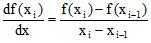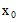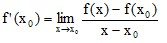# 2D curve: derivative

## Introduction

The user can represent the result of derivatives calculated from functions of spatial quantities or input/output parameters in the form of curves.

## Operation

The curves of derivatives are created as follows:

Stage Description
1 The Derivative of a 2D curve command is applied to an elementary curve or a set of elementary curves of the 2D curve entity.
2 The function representing the derivative of each analyzed curve is constructed by the calculation of the derivative at each point of the interval of the curve definition.
3 The new elementary curves are added to the already available curves of the 2D curve entity.

## Principle

The derivative is calculated starting from the function represented by the analyzed curve. The backward derivative principle is used.

Given a curve representing the function f(x), the calculation of the derivative in each point is carried out as follows:The number of points of the curve representing the derivative is the same as that of the initial curve minus 1.

Thus:

The 1st abscissa of the curve representing the derivative corresponds to the 2nd abscissa of the initial curve; the 2nd abscissa of the curve representing the derivative corresponds to the 3rd abscissa of the initial curve, etc.

The abscissa values must be strictly increasing ones (b>a).

## Backward derivative: reminder

The backward derivative of the function f inis equal to:## Calculate the derivative of a 2D curve

To calculate (and plot) the derivative of a 2D curve, follow instructions below:

Step Action
1

In the menu Curve > 2D curve (…):

• click on Derivative of a 2D curve
A selection box opens
2

In the selection dialog:

• choose the 2D curve entity
3

In the Derivative of a 2D curve dialog:

• choose the elementary curve(s) to be analyzed
The curves of the derivative are calculated and displayed in the 2D curve sheet.